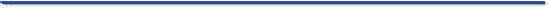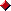The JavaTM TutorialTrail: Learning the Java Language
Lesson: Language Basics

## Shift and Logical Operators

A shift operator performs bit manipulation on data by shifting the bits of its first operand right or left. The next table summarizes the shift operators available in the Java programming language.

Shift Operators
Operator Use Description
`<<` `op1 << op2` Shift bits of `op1` left by distance `op2`; fills with zero bits on the right-hand side
`>>` `op1 >> op2` Shift bits of `op1` right by distance `op2`; fills with highest (sign) bit on the left-hand side
`>>>` `op1 >>> op2` Shift bits of `op1` right by distance `op2`; fills with zero bits on the left-hand side

Each operator shifts the bits of the first operand over by the number of positions indicated by the second operand. The shift occurs in the direction indicated by the operator itself. For example, the following statement shifts the bits of the integer 13 to the right by one position:

```13 >> 1;
```
The binary representation of the number 13 is 1101. The result of the shift operation is 1101 shifted to the right by one position — 110, or 6 in decimal. The left-hand bits are filled with 0s as needed.

The following table shows the four operators the Java programming language provides to perform bitwise functions on their operands:

Logical Operators
Operator Use Operation
`&` `op1 & op2` Bitwise `AND` if both operands are numbers;
conditional `AND` if both operands are boolean
`|` `op1 | op2` Bitwise `OR` if both operands are numbers;
conditional `OR` if both operands are boolean
`^` `op1 ^ op2` Bitwise exclusive `OR` (`XOR`)
`~` `~op2` Bitwise complement

When its operands are numbers, the `&` operation performs the bitwise `AND` function on each parallel pair of bits in each operand. The `AND` function sets the resulting bit to 1 if the corresponding bit in both operands is 1, as shown in the following table.

The Bitwise AND Function
Bit in `op1` Corresponding Bit in `op2` Result
000
010
100
111

Suppose that you were to `AND` the values 13 and 12, like this: `13 & 12`. The result of this operation is 12 because the binary representation of 12 is 1100, and the binary representation of 13 is 1101.

```    1101     //13
& 1100     //12
------
1100     //12
```
If both operand bits are 1, the `AND` function sets the resulting bit to 1; otherwise, the resulting bit is 0. So, when you line up the two operands and perform the `AND` function, you can see that the two high-order bits (the two bits farthest to the left of each number) of each operand are 1. Thus, the resulting bit in the result is also 1. The low-order bits evaluate to 0 because either one or both bits in the operands are 0.

When both of its operands are numbers, the `|` operator performs the inclusive or operation, and `^` performs the exclusive or (`XOR`) operation. Inclusive or means that if either of the two bits is 1, the result is 1. The following table shows the results of an inclusive or operation.

The Bitwise Inclusive OR Function
Bit in `op1` Corresponding Bit in `op2` Result
000
011
101
111

Exclusive or means that if the two operand bits are different the result is 1; otherwise the result is 0. The following table shows the results of an exclusive or operation.

The Bitwise Exclusive OR (XOR) Function
Bit in `op1` Corresponding Bit in `op2` Result
000
011
101
110

And finally, the complement operator (`~`) inverts the value of each bit of the operand: If the operand bit is 1, the result is 0; if the operand bit is 0, the result is 1. For example, `~1011` (11) is `0100` (4).

Among other things, bitwise manipulations are useful for managing sets of boolean flags. Suppose, for example, that your program had several boolean flags that indicated the state of various components in your program: is it visible, is it draggable, and so on. Rather than define a separate boolean variable to hold each flag, you could define a single variable, `flags`, for all of them. Each bit within `flags` would represent the current state of one of the flags. You would then use bit manipulations to set and to get each flag.

First, set up constants that indicate the various flags for your program. These flags should each be a different power of 2 to ensure that each bit is used by only one flag. Define a variable, `flags`, whose bits would be set according to the current state of each flag. The following code sample initializes `flags` to 0, which means that all flags are `false` (none of the bits are set):

```static final int VISIBLE = 1;
static final int DRAGGABLE = 2;
static final int SELECTABLE = 4;
static final int EDITABLE = 8;

int flags = 0;
```
To set the visible flag when something became visible, you would use this statement:
```flags = flags | VISIBLE;
```
To test for visibility, you could then write:
```if ((flags & VISIBLE) == VISIBLE) {
...
}
```
Here's the complete program, `BitwiseDemo`, that includes this code.
```public class BitwiseDemo {

static final int VISIBLE = 1;
static final int DRAGGABLE = 2;
static final int SELECTABLE = 4;
static final int EDITABLE = 8;

public static void main(String[] args) {
int flags = 0;

flags = flags | VISIBLE;
flags = flags | DRAGGABLE;

if ((flags & VISIBLE) == VISIBLE) {
if ((flags & DRAGGABLE) == DRAGGABLE) {
System.out.println("Flags are Visible "
+ "and Draggable.");
}
}

flags = flags | EDITABLE;

if ((flags & EDITABLE) == EDITABLE) {
System.out.println("Flags are now also Editable.");
}
}
}
```
Here's the output from this program:
```Flags are Visible and Draggable.
Flags are now also Editable.
```